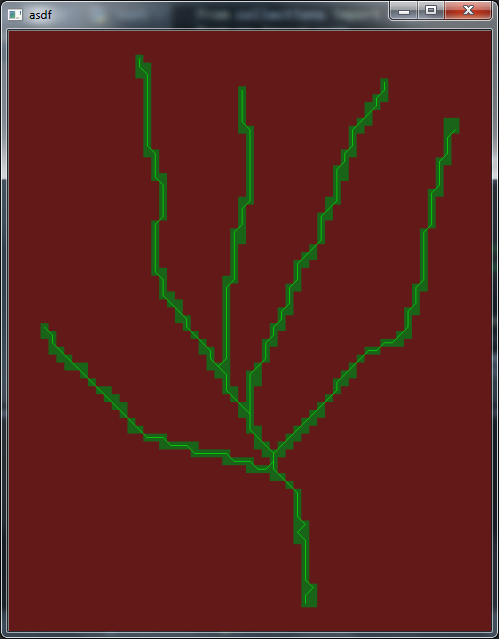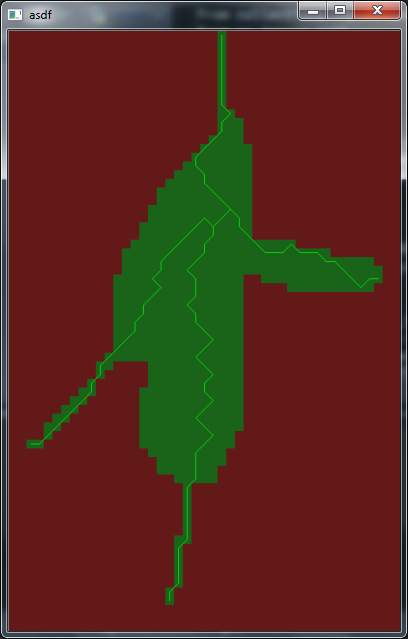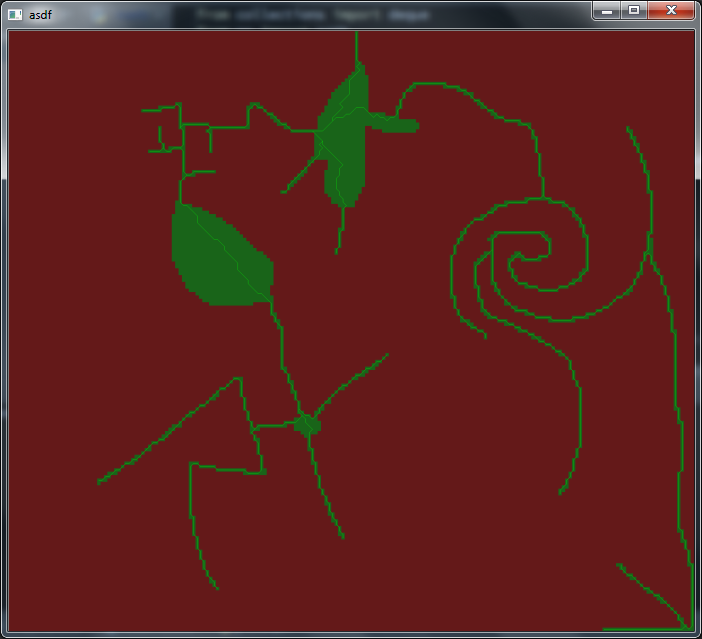# Building tree/graph from image points

I start to describe my problem with this picture:In the picture, we can see some points (black dots). What I want to do is to first store all the points and then find the node points and the tip points (red dots). What is more, I need to check if these red points can be connected by straight lines (along the black points) to find angles between the red lines.

I don't know if I explained it clearly enough, but what I figured is that I should implement a tree/graph and than use some path finding to check if the red points are connected?

Basically, I started with something like:

``````class Point {
public:
int x;
int y;
vector<Point> neighbors;

Point(void);
Point(int x, int y);
}

vector<Point> allPoints;
``````

Where I store all the points in `allPoints` vector. Than for each `Point`, I check all his neighbors ([x+1,y], [x-1,y], [x+1,y+1], [x-1, y+1], ...) and store them in neighbors vector for that `Point`.

Then, by the size of the neighbors vector, I determine if the Point is a node (3 or more neighbors), a tip (1 neighbor), or just some basic point (2 neighbors).

And here comes the part, where I have no idea how to implement some path finding (to check whether there is a way for example from a tip point to the closest node point).

What is more, I have no idea if my "tree" representation is good (probably is not). So if anyone would help me to achieve what I want, it would be great.

P.S. I'm writing in C++ (and OpenCV) and VS2010.

Edit:

This is how it looks like in a real program (red lines are drown by me in paint, but this is what i want to achieve):• Do you have some existing data structure that identifies the neighbor vectors? Or do you just a have a set of x/y points at the beginning? Are the branches always cleanly isolated like in the image? Or could it be more messy? Can you have cycles or re-connecting branches (a graph) or can you say that you will not allow cycles (a tree)? It seems like the problem needs a more detailed specification. Dec 22, 2013 at 14:17
• Do you know where to start(the root of the tree?) Dec 22, 2013 at 14:39
• So: I have just a set of x/y points at the beginning. Lets say that braches are cleanly isolated, I will not allow cycles (it would be a tree). And do I know where to start? Well, what if I don't? I believe (possibly wrong) that it doesn't matter which of the "tip" points will be the root. Dec 22, 2013 at 15:07
• There isn't much to go on here (code) but this is an interesting problem. I'll work on it when I have time and post an answer if I come up with something. Dec 23, 2013 at 0:45
• One approach that comes to mind: start from tips and follow the neighbors (which are basic points) until arriving at a node. If it arrives at the node, there's a path from that node to the point. Dec 23, 2013 at 7:06

I'm not sure if that post should be an answer or edit, because i have no idea if anyone would notice that I added something, co i decided to post it as an answer. Sorry if its wrong.

About a problem. I did some codding, I belive I know how to do what I wanted, but another problem came out ;d.To see where is a problem, you have to zoom above picture, about 400%, and take a look inside green rectangles. Image "skeleton" are my basic lines, and image "temp", are my output tips and nodes. As you can see, tips are fine (violet rectangles) (points with 1 neighbor) but unfortunately there are lines where pixel have 3 or more neighbors and they are not nodes (right green rectangle on "skeleton" is the line which have no nodes.. and green rectangle on "temp" are my false nodes .. marked because of the specific pixels positions).When You super zoom it, You are going to notice that I marked pixels with colors to make it more clear.

So the problem is that sometimes both nodes and "branches" have more than 2 neighbors which leads me to a problem "how to find a difference between them". All i need are nodes (small green rectangle on "skeleton" image - when you zoom you will see that there are 2 pixels that could be a node, but this is not important as long as they are so close to each other).

Any help?

If you need code, just tell and I will edit & paste it.

Edit:

I did something!

I found a way to filter redundant pixels from the lines. But that made some of my skeleton lines to disconnect, and that is not good, because some nodes are considered as "tips" now.

Just look at the picture:Small dots are "good" nodes, but dots inside red rectangles, are nodes which got disconnected (zoom in to see that), and are now considered as tips.

How did I filtered the pixels? Here is the code:

``````void SKEL::clearPixels(cv::Mat& input)
{
uchar* data = (uchar *)input.data;
for (int i = 1 ; i < input.rows-1 ; i++)
{
for (int j = 1 ; j < input.cols-1 ; j++)
{
if (input.at<uchar>(i,j) == 255) //if its part of skeleton
{
if (input.at<uchar>(i-1,j+1) == 255)
{
if (input.at<uchar>(i,j+1) == 255)  data[i*input.step+(j+1)*input.channels()] = 0;
if (input.at<uchar>(i-1,j) == 255)  data[(i-1)*input.step+(j)*input.channels()] = 0;
}
if (input.at<uchar>(i+1,j+1) == 255)
{
if (input.at<uchar>(i,j+1) == 255)  data[i*input.step+(j+1)*input.channels()] = 0;
if (input.at<uchar>(i+1,j) == 255)  data[(i+1)*input.step+(j)*input.channels()] = 0;
}
if (input.at<uchar>(i-1,j-1) == 255)
{
if (input.at<uchar>(i,j-1) == 255)  data[i*input.step+(j-1)*input.channels()] = 0;
if (input.at<uchar>(i-1,j) == 255)  data[(i-1)*input.step+(j)*input.channels()] = 0;
}
if (input.at<uchar>(i+1,j-1) == 255)
{
if (input.at<uchar>(i,j-1) == 255)  data[i*input.step+(j-1)*input.channels()] = 0;
if (input.at<uchar>(i+1,j) == 255)  data[(i+1)*input.step+(j)*input.channels()] = 0;
}
}
}
}
}
``````

For each pixel I checked if it has (x+1,y-1) (x+1,y+1) (x-1,y+1) (x-1, y-1) neighbor and if it does , i checked if there are neighbors just next to that neighbor and removed them. It was the only idea that I had , and its quite slow, but for now , nothing better comes to my mind.

So now, my main problem is. How to reconnect broken nodes ? ..

• It's good you are making progress. I am still working on it little by little when I have time. Since you have code that is working, you might have luck getting it reviewed on CodeReview? It sounds like your problem is lines that are not exactly one pixel. That is difficult. Also it looks like there are broken parts of the line. Is that right? Dec 27, 2013 at 1:12
• Well the biggest problem, are the lines that are no exactly one pixel, that's true. If You mean that lines on "temp" are broken - they are not, I just didn't draw all of them on purpose, to easier see where the multiple-pixel lines are exactly :P I came out with some idea, i will try it out today, and post the results. Dec 27, 2013 at 11:51
• Did you get something that works? I've actually tried about 5 different algorithms and they work ok but are not robust to various inputs. Jan 7, 2014 at 4:10

This is late but I got something working and put it in a github repo: pixeltree (it's too big to just paste the whole thing here). I work in python rather than c++ but I hope the idea will help if you or someone else needs it. Disclaimer - there is probably some accepted method of doing this but I didn't find it.

# Approach

1. Separate the image into as many regions as you want (e.g. black/white)
2. For each region, choose any pixel
3. Build a full tree from that pixel that covers every pixel in the region
4. Cut back the non-meaningful branches of the tree until it is a minimal tree

# Details

The hard part is the last step of cutting back the tree. This is the approach I used:

1. For each region again, choose a root at a "distant" pixel (technically, one end of the longest path in the graph).
2. For each leaf in that tree, perform a breadth-first-search until encountering another branch. Eliminate the branch of this leaf if its height is less than the encountered pixel.

# Examples# Embarrassingly large function that does most of the work:

``````def treeify(image, target_families=None):
# family map is used everywhere to identify pixels, regions, etc.
family_map = _make_family_map(image)
target_families = target_families or np.unique(family_map)
trees = list()
rows, cols = family_map.shape
remaining_points = set((r, c) for r in range(rows) for c in range(cols)
if family_map[r, c] in target_families)
# the "tree" here is actually just a non-cyclic undirected graph which could
# be rooted anywhere. The basic graph is done with each pixel pointing to some neighbors (edges)
edges = np.empty_like(family_map, dtype=object)
edges.flat = [set() for _ in edges.flat]
# continue until all regions within the graph are handled
while remaining_points:
# grow a tree from any remaining point until complete
start_p = remaining_points.pop()
family = family_map[start_p]
tree = {'family': family,
'any_point': start_p}
trees.append(tree)
q = [(None, start_p)]
while q:
# pushright + popleft --> breadth first expansion
# random within left part of q - roughly BFS with less pattern
source, p = q.pop(random.randrange(0, max(1, len(q)//2)))
try:
remaining_points.remove(p)
except KeyError:
pass # tree start point is always already gone
# send qualifying neighbors for expansion
q_points = tuple(qp for sp, qp in q)
expansion_points = [n for n in _neighbors(p, 'all', family_map)
if all((n != source,
n in remaining_points,
n not in q_points,
family_map[n] == family))]
expansion_pairs = [(p, n) for n in expansion_points]
q.extend(expansion_pairs)
# document all edges for this point
if source is None:
all_connections = list(expansion_points)
else:
all_connections = expansion_points + [source]
edges[p].update(all_connections)

# prune all but "best" branches within each area
for tree in trees:
family = tree['family']
# root graph at one end of the longest path in the graph
distant_point = _most_distant_node(tree['any_point'], edges)
# for choosing best paths: document the height of every pixel
heights = _heights(distant_point, edges)
remaining_leaves = set(_leaves(distant_point, edges))
# repeatedly look for a leaf and decide to keep it or prune its branch
# stop when no leaves are pruned
while remaining_leaves:
leaf = remaining_leaves.pop()
# identify any degenerate path to next branching pixel
# this path is ignored when testing for nearby branches
ignore = set(_identify_degenerate_branch(leaf, edges))
# BFS expansion to find nearby other branches
expansion_q = deque()
expansion_q.append(leaf)
while expansion_q:
p = expansion_q.popleft() # pushright + popleft for BFS
# decide what to do with each neighbor
for n in _neighbors(p, 'sides', family_map):
if n in ignore:
continue # already decided to ignore this point
elif n in expansion_q:
continue # already slated for expansion testing
elif family_map[n] != family: